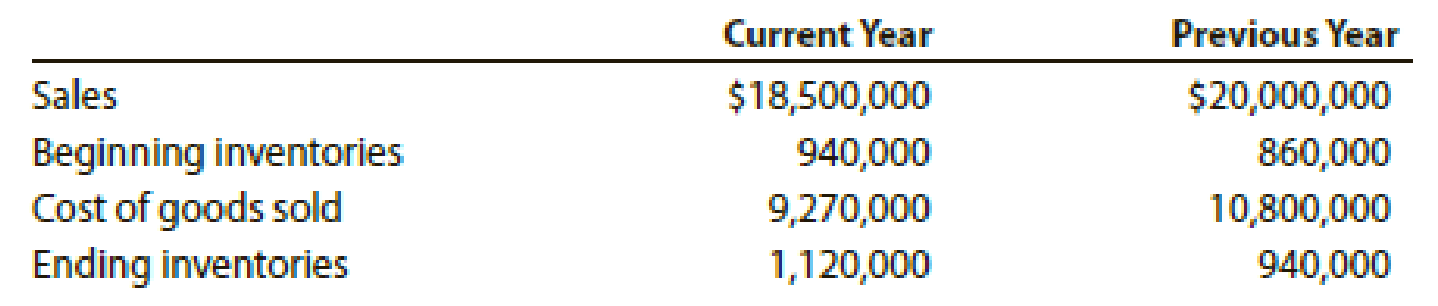# Inventory analysis The following data were extracted from the income statement of Keever Inc.: a. Determine for each year (1) the inventory turnover and (2) the number of days’ sales in inventory. Round to the nearest dollar and one decimal place. b. What conclusions can be drawn from these data concerning the inventories?### Financial Accounting

15th Edition
Carl Warren + 2 others
Publisher: Cengage Learning
ISBN: 9781337272124

#### Solutions

Chapter
Section### Financial Accounting

15th Edition
Carl Warren + 2 others
Publisher: Cengage Learning
ISBN: 9781337272124
Chapter 17, Problem 11E
Textbook Problem
31 views

## Inventory analysisThe following data were extracted from the income statement of Keever Inc.:a. Determine for each year (1) the inventory turnover and (2) the number of days’ sales in inventory. Round to the nearest dollar and one decimal place. b. What conclusions can be drawn from these data concerning the inventories?

a)

To determine

Compute inventory turnover ratio and number of days’ sales in inventory.

### Explanation of Solution

Financial Ratios: Financial ratios are the metrics used to evaluate the liquidity, capabilities, profitability, and overall performance of a company.

Inventory turnover ratio is used to determine the number of times inventory used or sold during the particular accounting period.

Formula:

Inventory turnover=Cost of goods soldAverage inventory

Number of days’ sales in inventory is determined as the number of days a particular company takes to make sales of the inventory available with them.

Formula:

Number of days’ sales in invenotry=Average inventory Average daily cost of goods sold

(1)

Compute inventory turnover ratio for current year.

Inventory turnover=Cost of goods soldAverage inventory=$9,270,000$1,030,000=9.0times

Compute inventory turnover ratio for previous year.

Inventory turnover=Cost of goods soldAverage inventory=$10,800,000$900,000=12.0times

Hence, the inventory turnover ratio for current year and previous year is 9.0 times and 12.0 times.

(2)

Compute number of days’ sales in inventory for current year.

Number of days’ sales in inventory=Average inventory Average daily cost of goods sold=$10,30,000$25,397=40

b)

To determine

Conclude Position of inventory.

### Still sussing out bartleby?

Check out a sample textbook solution.

See a sample solution

#### The Solution to Your Study Problems

Bartleby provides explanations to thousands of textbook problems written by our experts, many with advanced degrees!

Get Started